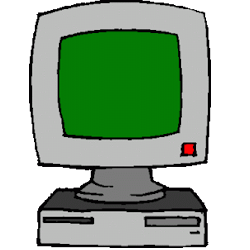Games
Problems
Go Pro!

# Understanding Computer Variables

Lesson Plans > Technology > Software > Programming

## Understanding Computer VariablesYou spend weeks teaching your students about variables--the most important fundamental they'll need to get anywhere in algebra. Then they go to computer class, and have to learn all over again what a variable is. Although we call them the same thing, and we give them the same names (like x and y) computer variables and algebra variables are not the same thing. If you don't believe this, consider the following statement, which is perfectly legitimate if you are a computer programmer, but has no solution if you are a mathematician:

I = I + 1

If you are a mathematician, this statement suggests that there might be a number out there which is equal to one more than itself. No such luck. But if you are a computer programmer, it means, 'Start with a certain value, and add one to it.' A perfectly legal computer instruction.

So how do you help your students understand the logic of computer variables? Here is one possible class activity.

For this activity, you will need beads, or marbles, or some other small object, and a desk organizer (one of those cabinets with little drawers you can put paperclips and thumbtacks in). For the purpose of the demonstration, you will label each drawer with a letter (X, Y, Z, etc).

Explain to the students that the drawers represent variables. This is a reasonable comparison since--unlike an algebraic variable--a computer variable is nothing more than 'a place to store something'--specifically, it is a place to store a value. The value stored in each variable will be represented by the marbles.

In the beginning, you can explain, each variable is empty because you haven't stored anything in it.

Now show your students a simple snippet of code like the following:

X = 7
Y = 2
Z = X - Y
Y = X - 6
Y = Y + 1

Talk through this piece of code as described below. Note that actions are marked in bracketed italics.

The first instruction says to store 7 marbles in drawer X. [put 7 marbles in drawer X]

The second instruction says to store 2 marbles in drawer Y. [put 2 marbles in drawer Y]

The third instruction says to find out how many marbles are in drawer X, [open drawer X again, and count the marbles. Write this down] and subtract the number of marbles in drawer Y [open drawer Y and count the marbles, write it down] and put that many marbles in drawer Z. [7 - 2 = 5. put 5 marbles in drawer Z]

The fourth instruction says to find out how many marbles are in drawer X [once again, count how many marbles are in drawer X. Write it down], subtract six from that [write out the calculation 7 - 6 = 1] and put that many marbles in drawer X. [Count the marbles in drawer Y] Oh, wait a minute, this drawer already has marbles in it. We need to take them out, and put in one. [take out all marbles, put one in]

The last instruction says to find out how many marbles are in drawer Y [Count the marbles in drawer Y] and add one. And that's how many marbles we're supposed to have in drawer Y. [Empty drawer Y, then put 2 marbles in]

Once you have walked through this process, manually putting marbles in and taking them out, have other students try. Write code snippets down and have students 'act them out'. After going through several examples, give exercises for students to try just using pencil and paper.
Lesson by Mr. Twitchell# Blogs on This SiteReviews and book lists - books we love!The site administrator fields questions from visitors.Like us on Facebook to get updates about new resources